# Pre-Algebra : Integers and Types of Numbers

## Example Questions

← Previous 1 3 4 5

### Example Question #1 : Number Theory

Which of the following is an integer and is not a whole number?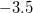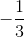Explanation:

An integer is any number without a fractional component, such as the following: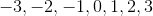A whole number is any positive number with no fractional component, such as the following: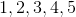The major difference between the two groups of numbers is that there are no negative whole numbers, while there are negative integers.

The first step of this problem is to eliminate any choices that are not integers. The choices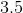, and, are all fractions or contain fractional components, so none of these values can be considered integers.

Of the remaining choices,falls under the categories of whole number and integer, so it cannot be the answer.fufills both requirements of the question. It is an integer by having no fractional component, and it is not a whole number because it is a negative value, so it must be the correct answer.

### Example Question #1 : Integers And Types Of Numbers

Which of the following is not a natural number?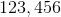Explanation:

Natural numbers are considered the "counting numbers". They are all of the integers from 1 until infinity. 0 and negative integers are not natural numbers, therefore, the answer to this problem is.

### Example Question #12 : Number Theory

Which of the following describes the number?

whole number

all of the other choices

natural number

integer

integer

Explanation:

Natural numbers are the counting numbers fromto infinity (all positive numbers). Whole numbers are the natural numbers plus. Integers are the positive and negative counting numbers, including. Therefore, the most accurate term to describe the numberis an integer.

### Example Question #11 : Number Theory

Which of these numbers is an integer but not a whole number?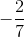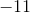Explanation:

The whole numbers are the elements of the set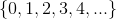.

The integers are the the elements of the set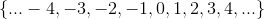.

The numbers that belong to the former but not the latter are exactly the set of negative integers. Of the given choices, onlyis such a number. It is the correct choice.

### Example Question #1 : Integers And Types Of Numbers

Evaluate: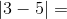Explanation:

First evaluate everything inside of the absolute value. 3-5=-2.

The absolute value then turns all negative numbers into a positive number, so the -2 becomes a positive 2.

### Example Question #1 : Properties Of Integers

If a and b are even integers, what must be odd?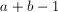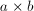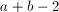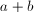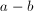Explanation:

The sum (or difference) or 2 even integers is even. Similarly, the product (or quotient) of 2 even integers is also even; therefore the answer must be, which can be easily checked by plugging in any two even numbers.

For example, if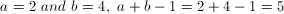, which is odd.

### Example Question #1 : Understanding The Properties Of Integers

What is the greatest prime factor of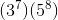Explanation:

The only prime factors are 3 and 5, therefore, 5 will be the greatest prime factor.

### Example Question #1 : Integers And Types Of Numbers

Isan integer, decimal, or a fraction?

Decimal

Fraction

None

Integer

Fraction

Explanation:

A fraction is a number with a numerator and denominator that can not be simplified into an integer so the numberis a fraction.

### Example Question #1 : Integers And Types Of Numbers

Which of the following is an integer?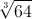-100.1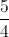1.5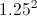Explanation:

Integers are any number that doesn't have anything in the decimal places.  They are also known as whole numbers.  The cubed root of 64 is 4, which is the only whole number out of the answer choices.

### Example Question #55 : High School Math

Which of the following is NOT an integer?

1024

-4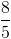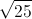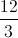An integer is simply a whole negative or positive number, which means it can be expressed without decimal places.  Any number can be considered an integer as long as it fits this criteria.  They can be expressed as square roots, fractions, or anything else, as long as the final answer is a whole number.  In this question, the only answer that is not a whole number is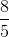, which is 1.6.